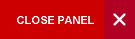## Introduction to Semiconductor Devices

Semester 2

This course provides the basic foundation for understanding electronic semiconductor devices and their circuit applications and limitations. It has introductory elements of quantum mechanics as a requirement for understanding the dynamics of the behavior of charge carriers and energy distributions within a semiconductor lattice and across p-n junctions. As such, reasonably strong mathematical and electrical field theory backgrounds are required – as obtained from the prerequisites.

The three fundamental areas of semiconductor devices; semiconductor theory, p-n junction devices and field effect devices, are adequately covered in this course. The learning experience is enhanced with computer-based exercises and assignments. The assignments will include: (1) a take home problem solving questions design to test student understanding of the theory; and (2) a report in the form of an IEEE paper structure on a survey of the state-of-the-art in semiconductor devices and manufacturing. The intent is to expose the student to high level technical publications. Circuit simulation tools will be used throughout this course.

Syllabus:

1.      Semiconductor Fundamentals

• General introduction to semiconductor; Carrier modeling, energy quantization and probability concepts; energy bands structure, density of states, statistical mechanics; Semiconductor in equilibrium; Carrier transport and excess carrier phenomenon; Carrier Modeling; Carrier Action; Basics of device fabrications.

2.      PN Junctions

• PN Junction electrostatics; PN Junction Diode, I-V Characteristics, small signal admittance, Transient response; Optoelectronic Devices; microwave diodes – tunnel, IMPATT, Gunn.
• Bipolar Junction Transistors (BJT): BJT fundamentals, static characteristics, dynamic response modeling- equivalent circuits, transient response.
• PNPN Devices: Silicon controlled rectifiers (SCRs); TRIACS, DIACS,
• Metal Semiconductor contacts and the Schottky Diode
• Circuit application examples for PN junction devices

3.      Field Effect Devices

• The JFET and the MESFET; The Metal Oxide Semiconductor Field Effect Transistor (MOSFET)-theory of operation, ID-VD relationships, Threshold considerations; Non Ideal MOSFETs, Modern FET structures
• Circuit application examples for Field Effect Devices
Evaluation:

One 2-hour final exam                          60%

One 1-hour in-course tests                                20%

Assignments                                                     20%

• Six take-home problem solving assignment of equal weighting (10%)
• One paper on a survey of the state-of-the-art in the analogue circuit designs (10%). Report will take the form of that required for an IEEE paper publication.
Learning Objectives:

After completing this course, students should be able to:

• Identify crystalline planes and direction in a crystal lattice in Miller Indices
• Outline the classification of solids as metals, semiconductors, and insulators and distinguish direct and indirect semiconductors
• Calculate the carrier concentration in intrinsic and extrinsic semiconductors
• Apply the Fermi-Dirac distribution function to determine the occupation and the carrier concentration of electron and hole states in a semiconductor
• Calculate the contact potential of a p-n junction and estimate the actual carrier concentration in the depletion region of a p-n junction in equilibrium
• Distinguish between the current conduction mechanisms in forward and reverse biased diodes and calculate the minority and majority carrier currents
• Identify the mechanism for current flow in PN junction devices
• Calculate the terminal parameters of a BJT in terms of the material properties and device structure and estimate the β of a BJT
• Calculate the threshold voltage of an ideal MOS capacitor and non-ideal MOS transistors
• Determine the electrical characteristics for optoelectronic devices (solar cells, LED, photo-transistors and opto-isolators
• Apply the equivalent circuits of diodes and transistors to various circuit design
• Use SPICE software to perform comparative analyses between different models for transistors and diode
• Use SPICE software to analyze transistor and diode circuit characteristics
P14A/(PHYS1411 and PHYS1412) and P14B/(PHYS1421 and PHYS1422) and ELET1400 and M08B/MATH0100, M08C/MATH0110 or Equivalent

Required Textbook:

“Semiconductor Device Fundamentals”by Robert F. Pierret, 2007

1.       “Electronics devices and Circuit Theory” – by Robert L. Boylestad and Louis Nashelsky, 2008

2.       Solid State Electronic Devices – 6th Ed; B.G. Streetman and S.K. Banerjee, 2006

Internet Resources:

Course Code:
ELET2420
Credits:
3 Credits
Level:
Level 2
Top of Page